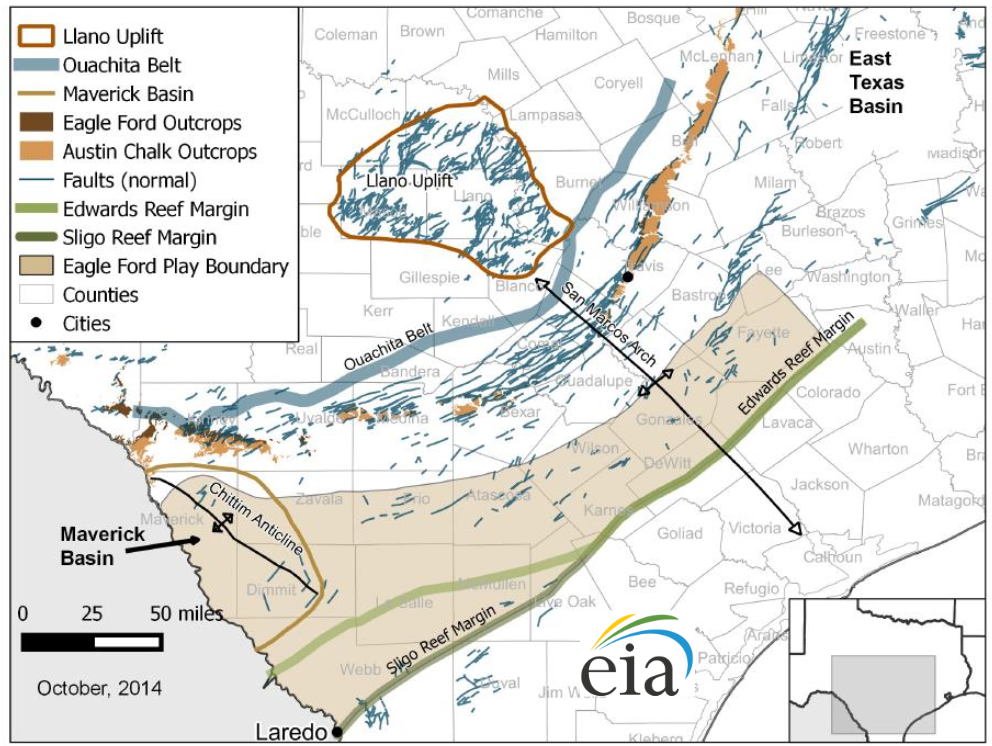# 5.6 Problems

1. For the following faults: (i) draw the corresponding points in a stereonet (lower hemisphere projection) map, and (ii) convert strike from quadrant to azimuth convention. Use https://app.visiblegeology.com/stereonetApp.html only to verify your answers.
1. N55E, 45SE
2. E20S, 60NE
3. N20W, 25SW
4. S10W, 60NW

2. Find out the ideal orientations of (a) a hydraulic fracture and (b) shear fractures, all created in a site subjected to stresseswherestrikes E-W,0.8, andis a principal stress.

3. Find out the ideal orientations of (a) a hydraulic fracture and (b) shear fractures, all created in a site subjected to stresseswherestrikes W20N,, andis a principal stress..

4. Find out the state of stress (regime and direction of stresses) that caused the faults mapped in the following stereonets:5. In a given reservoir under study, stresses are40 MPa,MPa,MPa,MPa, andacts E-W. For each of the faults below, calculate (using the 3D Mohr circle method if possible) the normal and shear stress on the plane of the fault and then determine if the fault is prone to slip assuming.
1. Fault with strike north-south, dip 65to the east
2. Fault with strike east-west, dip 35to the north
3. Fault with strike 060, dip 904. Fault with strike 030, dip 256. (Optional) Write a Matlab/Python code that can obtain (,,,) on an arbitrary fault with orientationfor a given state of stress.
1. Verify with solved problems in this chapter
2. Apply to solve all cases in Problem 5
3. Apply code to solve stress on 100 fractures randomly oriented with the stresses of Problem 5. Plot all results the respective 3D Mohr circle. A nearby injection wellbore will perform water flooding. What is the additional pore pressure needed to start reactivating faults?
Hint: Matlab function `rand' can be used for creating an array of random values;creates an array(Nx1) of values in interval7. Let us compare reservoirs with normal and reverse faulting regimes (onshore). For both regimes, plot the limits ofandversus depth from 0 to 5 km assuming lithostatic gradient equal to 23 MPa/km, hydrostatic pore pressure equal to 10 MPa/km, and a state of stress limited by frictional strength of faults. Assume sliding friction coefficient is0.6. For both regimes, how does changingfrom 0.6 to 1.0 change the maximum possible differential stressat a fixed depth ofkm?

8. A given site offshore (sea floor at 500 ft) is subjected to a Normal Faulting stress regime. Over-pressure exists and at 2,000 ft (TVDSS: True Vertical Depth Sub-Sea) overpressure is0.78. Calculate the total minimum horizontal stressat this depth (2,000 ft TVDSS) assuming frictional equilibrium of faults and friction angle 30. Draw the corresponding 2D Mohr circle with a solid line and draw an additional 2D Mohr circle with a dashed line assuming hydrostatic pressure. (Assume typical water and lithostatic gradients)

9. The following figure shows major faults in the Eagle Ford region [EIA, 2014 - Updates to the EIA Eagle Ford Play Maps].1. Looking at the normal faults in the Frio, Atascosa, and Wilson counties, what would be the expected direction of the minimum horizontal stressin this area?
2. Visit the GIS application of the railroad commission of Texas: https://gis.rrc.texas.gov/GISViewer/. Zoom into the South-East of the Frio county until you see well trajectories. The horizontal wells are expected to be drilled in the direction of the least principal stress. Does this direction ofcoincide with the direction expected from fault orientation? Justify.